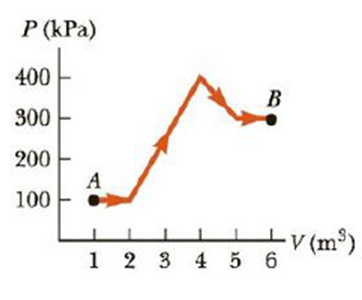# (a) Find the work done by an ideal gas as it expands from point A to point B along the path shown in Figure P12.8. (b) How much work is done by the gas if it compressed from B to A along the same path?### College Physics

11th Edition
Raymond A. Serway + 1 other
Publisher: Cengage Learning
ISBN: 9781305952300### College Physics

11th Edition
Raymond A. Serway + 1 other
Publisher: Cengage Learning
ISBN: 9781305952300

#### Solutions

Chapter
Section
Chapter 12, Problem 8P
Textbook Problem

## (a) Find the work done by an ideal gas as it expands from point A to point B along the path shown in Figure P12.8. (b) How much work is done by the gas if it compressed from B to A along the same path?Expert Solution

(a)

To determine
the work done by an ideal gas when it expands from point A to point B.

### Explanation of Solution

Given Info:

The width of rectangle A is 6m3 , the height of rectangle A is 100kPa , the base of triangle B is 2m3 , the height of triangle B is 300kPa , the width of the square D is 2m3 , the height of the square D is 200kPa , the base of triangle C is 1m3 , and the height of triangle C is 100kPa .

The area under the graph in a PV diagram is equal to the work done on the gas. If the final volume is greater than the initial volume, then the work done by the gas is positive.

Formula to calculate the area is,

A=(W1×H1)+12(B2×H2)+(W3×H3)+12(B4×H4)

• W1 is the width of rectangle A
• H1 is the height of rectangle A
• B2 is the base of triangle B
• H2 is the height of triangle B
• W3 is the width of square D
• H3 is the height of square D
• B4 is the
Expert Solution

(b)

To determine

To determine The work done by an ideal gas when it is compressed from B to A.

### Want to see the full answer?

Check out a sample textbook solution.See solution

### Want to see this answer and more?

Bartleby provides explanations to thousands of textbook problems written by our experts, many with advanced degrees!

See solution